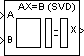# SVD Solver

Solve AX = B using singular value decomposition

•Libraries:
DSP System Toolbox / Math Functions / Matrices and Linear Algebra / Linear System Solvers

## Description

The SVD Solver block solves the linear system AX = B. The linear system can be overdetermined, underdetermined, or exactly determined. The block solves the system by applying singular value decomposition (SVD) factorization to the input matrix at the A port.

## Ports

### Input

expand all

Specify the input matrix A as an M-by-N matrix through this port. Inputs A and B must have the same number of rows.

Data Types: `single` | `double`
Complex Number Support: Yes

Specify the matrix B in the AX = B equation as an M-by-L matrix or an M-by-1 vector.

When you specify an M-by-1 vector, the block treats the length-M unoriented vector input at port B as an M-by-1 matrix. Inputs A and B must have the same number of rows.

Data Types: `single` | `double`
Complex Number Support: Yes

### Output

expand all

The output at the X port is the N-by-L matrix X. The block computes X to minimize the sum of the squares of the elements of BAX (the residual).

When B is a vector, this solution minimizes the vector 2-norm of the residual. When B is a matrix, this solution minimizes the matrix Frobenius norm of the residual. In this case, the columns of X are the solutions to the L corresponding systems AXk = Bk, where Bk is the kth column of B, and Xk is the kth column of X.

X is known as the minimum-norm-residual solution to AX = B. The minimum-norm-residual solution is unique for overdetermined and exactly determined linear systems, but the solution is not unique for underdetermined linear systems. Thus when you apply the SVD Solver block to an underdetermined system, the block computes X to minimize the number of nonzero entries in X.

Data Types: `single` | `double`
Complex Number Support: Yes

The block returns a logical scalar value of `0` if the singular value decomposition calculation converges, and a logical scalar value of `1` if the singular value decomposition calculation does not converge.

Data Types: `Boolean`

## Parameters

expand all

Select to enable the E output port, which reports the failure to converge as one of the following:

• `0` — The singular value decomposition calculation converges.

• `1` — The singular value decomposition calculation does not converge.

If the singular value decomposition calculation fails to converge, the output at port X is an undefined matrix of the expected size.

## Block Characteristics

 Data Types `double` | `single` Direct Feedthrough `no` Multidimensional Signals `no` Variable-Size Signals `no` Zero-Crossing Detection `no`

## Version History

Introduced before R2006a